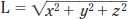# How is the length of a vector calculated?

The length of a vector is calculated by taking the square root of the sum of the squares of each coordinate. For example, if the vector is defined by (x, y, z), then the length (L) of the vector is calculated this way:Close

This is a web preview of the "The Handy Math Answer Book" app. Many features only work on your mobile device. If you like what you see, we hope you will consider buying. Get the App